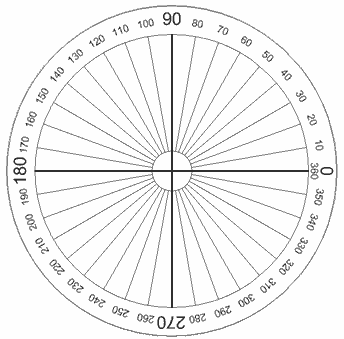# Find angle in degrees from one point to another in 2D space?

Given Point A and Point B in 2D space, how can I find the angle Point B is from Point A? 0° can be any direction; it doesn't matter. For example, Point A is at (0, 10) and Point B is at (10, 20). The angle is 45° in this example (assuming 0° is up).

I believe that the accepted answer does not correctly solve the problem for many people visiting this question. I am doing 2D simulations and the answer above does not solve my problem. Imagine the following circle:

$$\hskip1.8in$$Assume that the middle of the circle is point A. Point B is at some angle from A according to the angles of the circle (so 0°) is right.

$$\hskip2in$$The atan2 function is what you need!

$$atan2(y, x)$$ $$\hskip3.2in$$ Where

$$y = y_B - y_A$$ $$x = x_B - x_A$$

• You made my day. I spent hours trying to figure this out and got nothing but convoluted solutions that never return a proper angle. Thank you! Aug 28 '18 at 17:19
• Is x1,y1 point A, and x2,y2 point B? May 16 '20 at 15:21
• @SteveSmith yes. Jul 2 '20 at 12:21
• Maybe this is useful to someone: justingolden.me/angleto Mar 27 at 2:41

From what I understood about your question, you want to find the angle between two points given their coordinates. In that case, first find the slope of the line using the slope formula: $$m=\dfrac{y_2-y_1}{x_2-x_1}$$ where $(x_1,y_1)$ and $(x_2,y_2)$ are coordinates on the line. Next, use this formula: $$\tan(\theta)=m$$ where $\theta$ is the angle. Therefore, the angle $\theta$ equals: $$\theta=\tan^{-1}(m)$$

Let's use the points $(0,10)$ and $(10,20)$ as an example (you mentioned it in your question). The slope is: $$m=\dfrac{10-20}{0-10}$$ $$m=\dfrac{-10}{-10}$$ $$m=1$$ Now we will find $\theta$. $$\tan(\theta)=1$$ $$\theta=\tan^{-1}(1)$$ $$\theta=45^\circ$$
Note: The $\tan(\theta)=m$ formula only gives the angle facing the positive $x$-axis (i.e. facing the "right"). So for a negative slope, you should get an angle that is greater than $90^\circ$.

• In your example using my two points you used the Xs over the Ys. Is that intentional? Why does it differ from the equation above? Mar 14 '14 at 2:08
• @Keavon Nice observation, it is supposed to be the Ys over the Xs, sorry Mar 14 '14 at 3:19
• What about the scenario where A = (10, 0) and B = (10, 10), and so x2-y2 = 0 and you get a divide by 0 error? This isn't a paticularly helpful answer imo. Jul 15 '18 at 2:42
• Does this answer work if point B is to the left of point A? May 16 '20 at 15:24